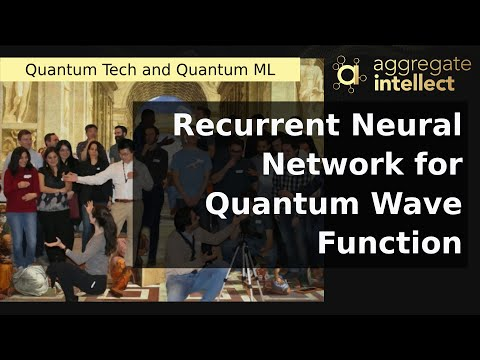Past Recording
Share
Star()
Recurrent Neural Network for Quantum Wave Function
Thursday Jul 30 2020 16:00 GMT
Please to join the live chat.###### Why This Is Interesting

The authors use recurrent neural networks (RNN) to estimate the ground state wavefunction ofg some quantum systems. RNN parameters are tuned to learn the approximate ground state of a quantum Hamiltonian using variational monte carlo. They leverage the autoregressive nature of this architecture for the efficient calculation of physical estimators by providing independent samples: ground state energies, correlation functions, and entanglement entropies for several quantum spin models of interest to condensed matter physicists in one and two spatial dimensions. RNNs are normally used for approximating classical distributions, say word probability distribution in natural language, but here they combine that with variational principle to approximate a quantum distribution, more specifically the amplitude and phase of the wavefunction.

###### Discussion Points
• How do symmetries of the physical system simulated here affected the architecture choices
• How is the system initialized? How is it trained? Do you start from an arbitrary state, calculate the variational energy, and then use your optimization method (vmc?) to tune your parameters and try again?
• In NLP people have moved on from RNN architectures to Transformers because of their higher flexibility and parallelisation, is that something that you envision happening as a future direction of your research?
###### Takeaways
• Neural network representation of the quantum state based on autoregressive models allow for uncorrelated sampling from the wavefunction, unlike traditional methods that usually introduce some sampling bias into the estimation
• You can map the lattice location in quantum many body systems to the time index of an RNN, to capture and iteratively / progresssively improve the estimation of the ground state by going through the folds of the RNN
• Generated wavefunctions can be used to estimate the energy of the system, and any optimization method (ADAM, VMC, …) can be used to tune the RNN parameters to obtain a better estimation of the ground state
• They used this approach to effectively calculate ground state energies, correlation functions, and entanglement entropies for several quantum spin models of interest to condensed matter physicists in one and two spatial dimensions
Time of Recording: Thursday Jul 30 2020 16:00 GMT